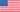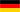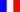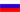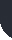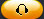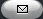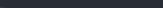Task Management Software for Team Collaboration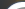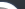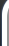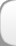HomeDownloadSupportPurchase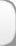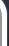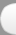Installation GuideStartUp GuideOnline Help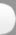# Cost of Goods Sold (COGS) by the Weighted Average Method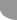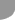Home  » Products  » VIP Task Manager Pro  » Tutorials  » Customization Guide  » Cost of Goods Sold (COGS) by the Weighted Average Method

Cost of Goods Sold (COGS) by the Weighted Average Method

There is an alternative way to calculate Cost of Goods Sold (COGS). The calculation is based on the average cost per unit of inventory for particular accounting period. The following formula is used to calculate Average Cost per Unit under the Weighted Average Method:

Average Cost per Unit = (Beginning Inventory + Purchases (in money)) ÷ (Beginning Inventory + Purchases (in units))

Beginning Inventory is the amount of inventory available for use or sale at the beginning of an accounting period. Beginning Inventory can be calculated by the formula:

Beginning Inventory = Number of beginning inventory units (or Quantity) x Cost per beginning inventory unit

Purchases (Inventory Purchases) is the total amount of purchases made over some period of time.

Inventory Purchases can be calculated by the formula below:

Inventory Purchases = Quantity of Purchases x Cost per unit of purchases

Having Average Cost per Unit it is possible to calculate:

1) Cost of Goods Sold (COGS) = Average Cost per Unit x Units Sold

2) End Inventory = Beginning Inventory in units (Quantity) x Average Cost per Unit

End Inventory is the amount of inventory available for use or sale in the end of an accounting period.

Using the Weighted Average Method every unit sold would have the same Average Cost per Units Sold irrespective of the actual cost this unit is sold. For example, you sell books. The weighted average cost is \$30.00 USD per book (\$4500 USD/150 books according to the formula above used to calculate Average Cost per Unit).  So the inventory price \$30 USD will be the same for each book bought, the actual price of one book is \$27 USD while another book was sold at \$32 USD. The Average Cost per units Sold is updated once the new purchase occurs.

If you need to calculate Cost of Goods Sold under the Weighted Average Method, please follow the steps below:

• Add custom field "Quantity" that will show the number of beginning inventory units
•• Add custom field "Cost per unit" to display the cost of beginning inventory per unit:
•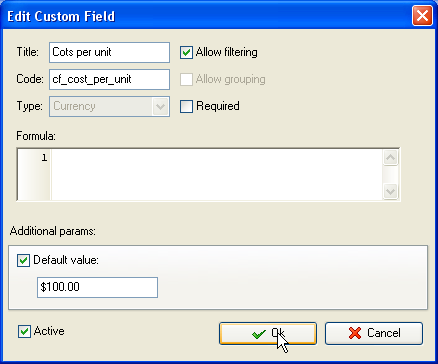• Add custom field "Beginning Inventory" to show the total cost of Beginning Inventory for a given accounting period.
•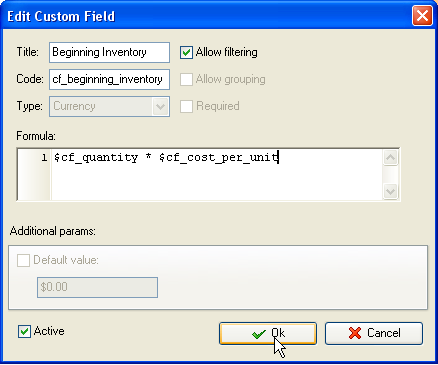• Create custom field "Quantity of Purchases" to show the number of purchases in units for a given accounting period:
•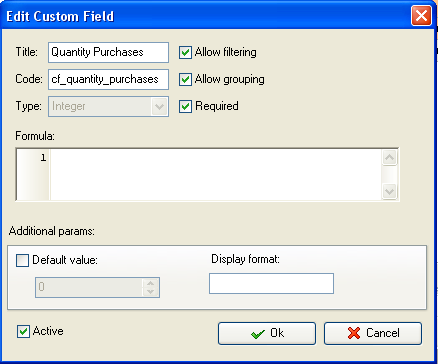• Add custom field "Cost per unit of purchases" to display the cost of each unit that was purchased within certain accounting period:
•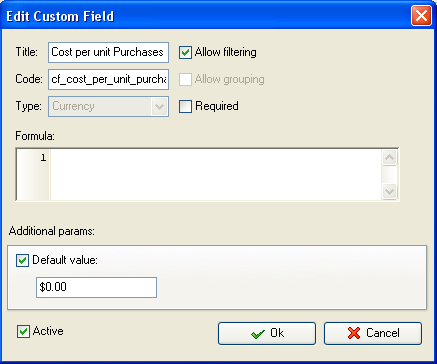• Create custom field "Inventory Purchases" to show the total cost of inventory purchased over accounting period:
•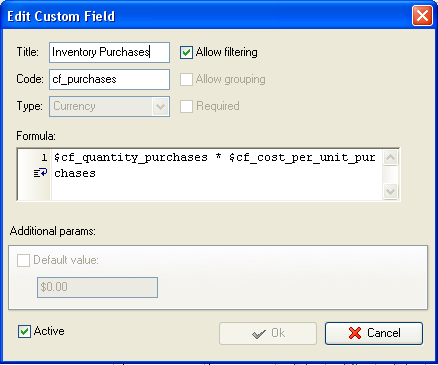• Create custom field "Average Cost per Unit". It will be calculated according to the formula: Average Cost per Unit = (Beginning Inventory + Purchases (in money)) ÷ (Beginning Inventory + Purchases (in units)) . The formula for custom field looks like this:
•         ToFloat(\$cf_beginning_inventory + \$cf_purchases) / ToFloat(\$cf_quantity + \$cf_quantity_purchases)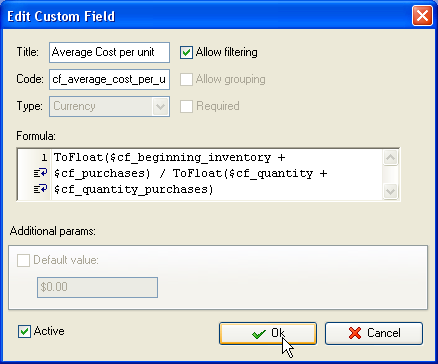• Add custom field "Cost of Goods Sold (COGS)":
•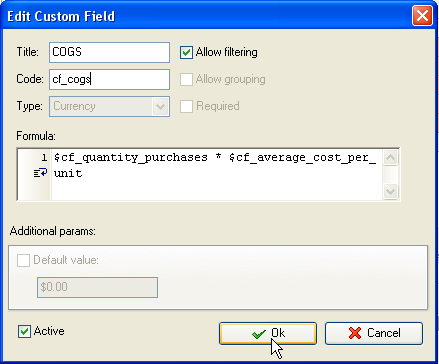• Add custom field "End Inventory". It is calculated based on Beginning Inventory in units (Quantity) and Cost of Goods Sold:
•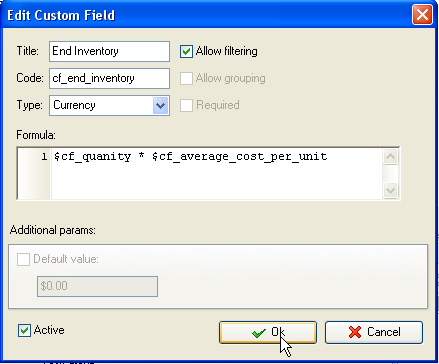• On Task Tree select task group for which you need to display Cost of Goods Sold and select "Custom fields options"
•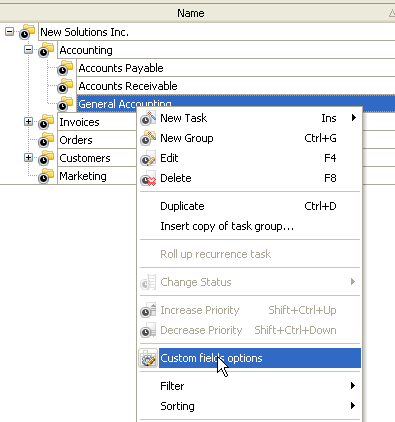• Set the following custom fields settings for the following custom fields : "Quantity", "Cost per unit", "Beginning Inventory", "Quantity of Purchases", "Quantity of Purchases" and "Inventory Purchases"
• In "Settings for" select "This task group"
• select "Enable"
• in "Aggregated (for task group)" field select "Sum" from drop-down list and the corresponding custom field name:
•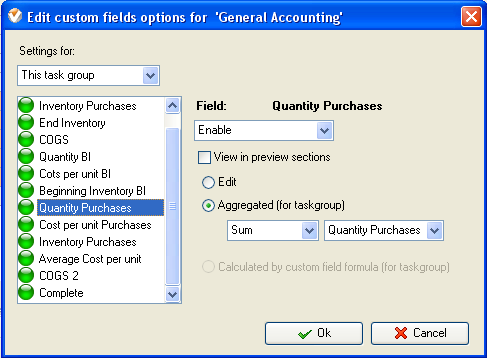• Select custom field "Average Cost per Unit", "COGS" and "End Inventory" set the following settings for these custom fields:
• In "Settings for" select "This task group"
• select "Enable"
• select "Calculated by custom field formula (for task group)"
•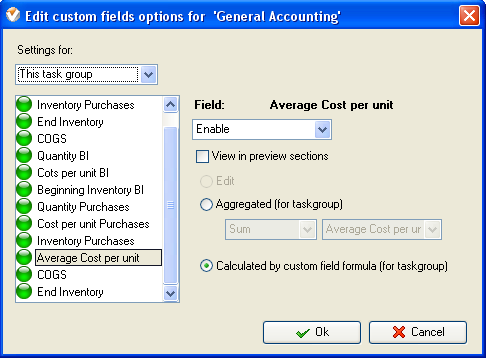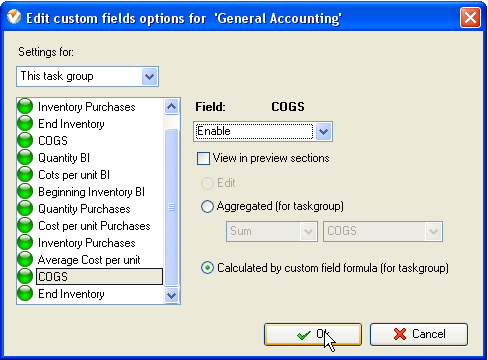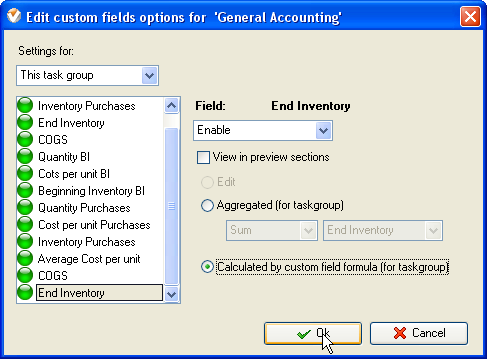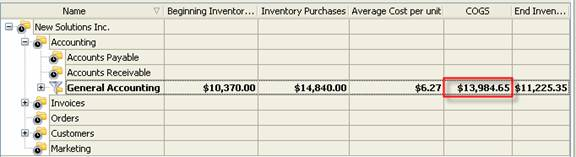Cost of Goods Sold (GOGS) under the Weighted Average Method on Task Tree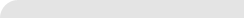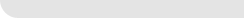» VIP Task Manager Pro VIP Task Manager Std Testimonials Turnkey Solution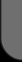ProductsSolutionsDownloadSupportPurchaseContactsForumVideoMy Account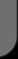Copyright © 2004 - 2023 Task Management Software